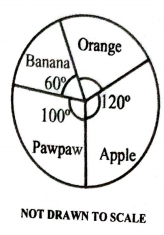mycollegehive
The pie chart represents fruits on display in a grocery sh...
waec-mathematics-2019 ssce-exam-2019 waec-2019 math statistics0The pie chart represents fruits on display in a grocery shop. If there are 60 oranges on display, how many apples are there?

A. 40

B. 80

C. 90

D. 70

188 viewsShareFollowUniversity of Lagos Nigeria
20 July 2020School not set Nigeria
07 August 20200The angle of orange is unknown but can be found since the sum of all angles in a pie chart must be 360. That is,

let x be the unknow angle of orange

60 + 100 + 120 + x = 360

280 + x = 360

Subtract 280 from both sides

x = 360 - 280

x = 80

Thus, the angle of orange is 80o

Since the number of oranges is given as 60, we can find that of apple by comparing the angle and number of apples with that of orangle.

let y be the number of apples displayed

That is,

80o/60 = 120o/y

cross multiply,

80y = 60 x 120

80y = 7200

divide both sides by 80

y = 7200/80

y = 90

Therefore, there are 90 apples on display

Ans CShare

### Groups

How to insert math formulas/equations### Related Tags

waec-mathematics-2019

4 followers

53 questionsssce-exam-2019

3 followers

64 questionswaec-2019

6 followers

64 questionsmath

15 followers

1261 questionsstatistics

0 followers

1 questions